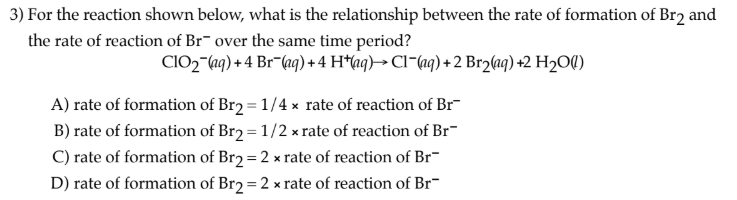# 3) For the reaction shown below, what is the relationship between the rate of formation of Br2 and the rate of reaction of Br- over the same time period? ClO2 -(aq) + 4 Br-(aq) + 4 H+(aq)→Cl-(aq) + 2 Br2(aq) +2 H2O(l) A) rate of formation of Br2 = 1/4 × rate of reaction of Br- B) rate of formation of Br2 = 1/2 × rate of reaction of Br- C) rate of formation of Br2 = 2 × rate of reaction of Br- D) rate of formation of Br2 = 2 × rate of reaction of Br-